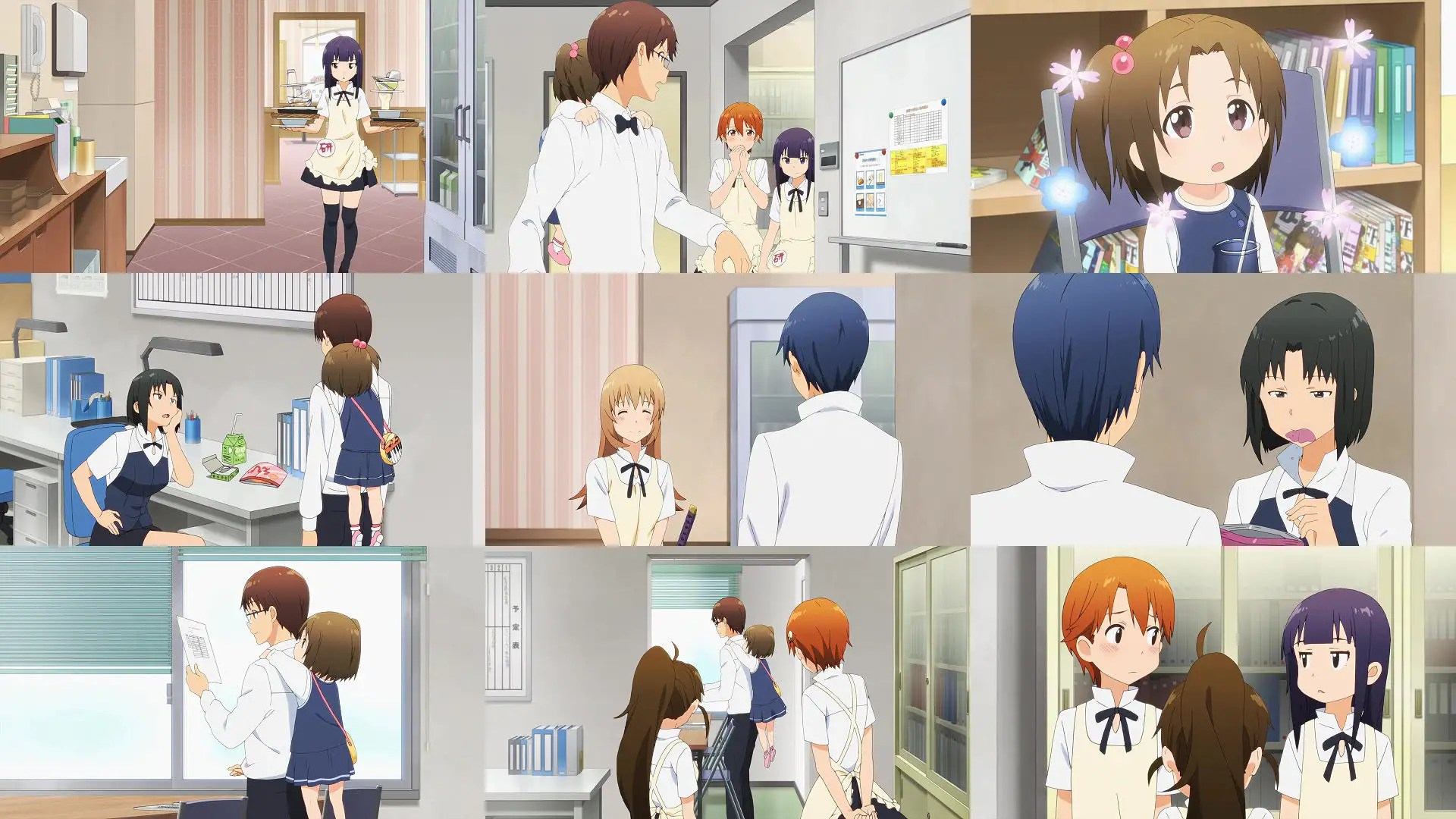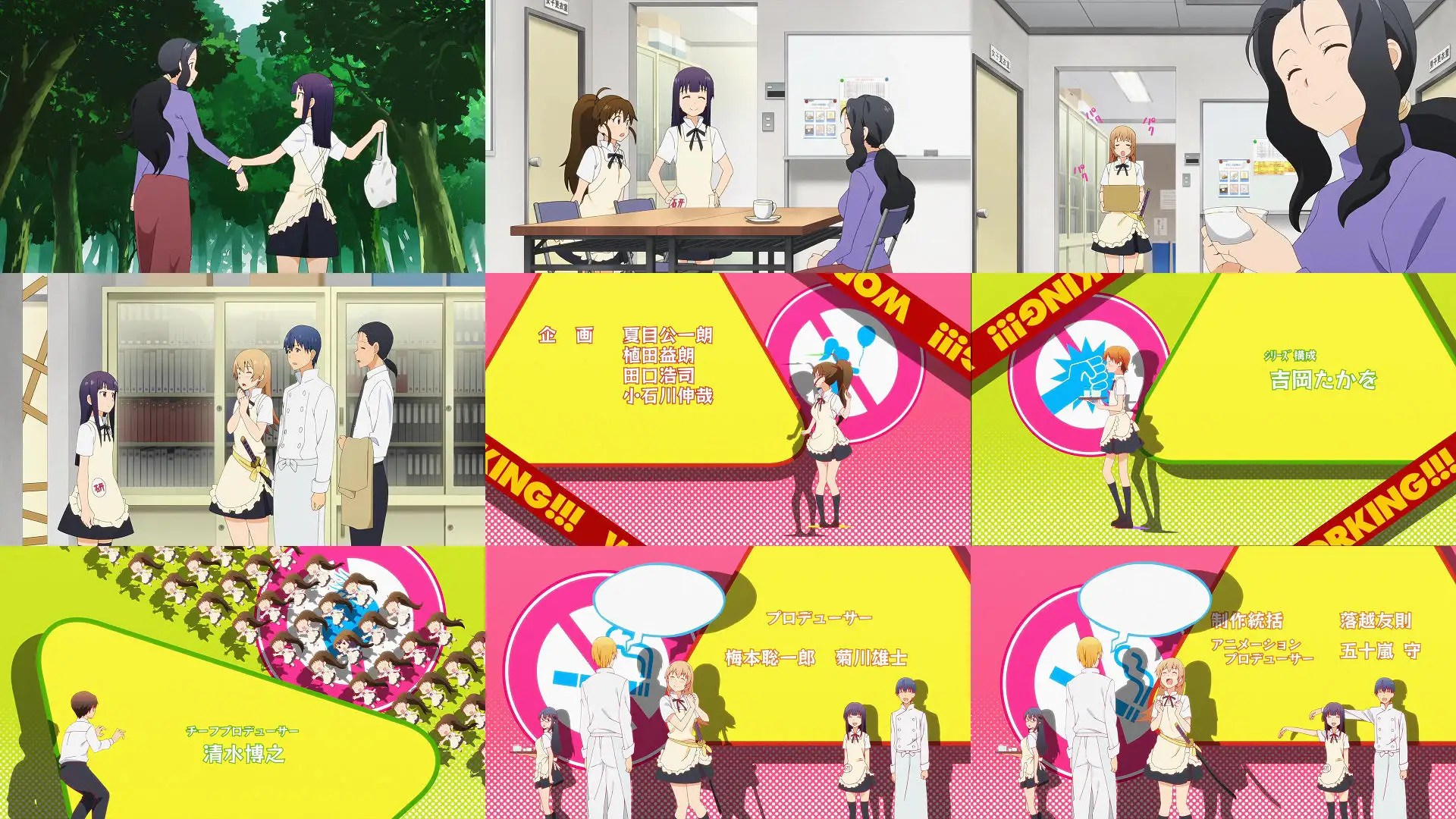三十代殘兵游勇

七月 6, 2015

20150706 七月夏季新番 其二 (六花の勇者；Charlotte；乱歩奇譚；城下町のダンデライオン；WORKING!!!…等等)

Filed under: 總合批評 — handelshieh @ 週一 2015/07/06 00:06:12 GMT+0800

「城下町のダンデライオン」「青春×機関銃」 「乱歩奇譚 Game of Laplace」「六花の勇者」「下ネタという概念が存在しない退屈な世界」 「 Charlotte」「GATCHAMAN CROWDS insight」「WORKING!!!」

4+3+3+3+4 4+3+3+3+3=33 「城下町のダンデライオン 001」各人王家能力介紹回。目前看來像是超能力家族喜劇的樣子。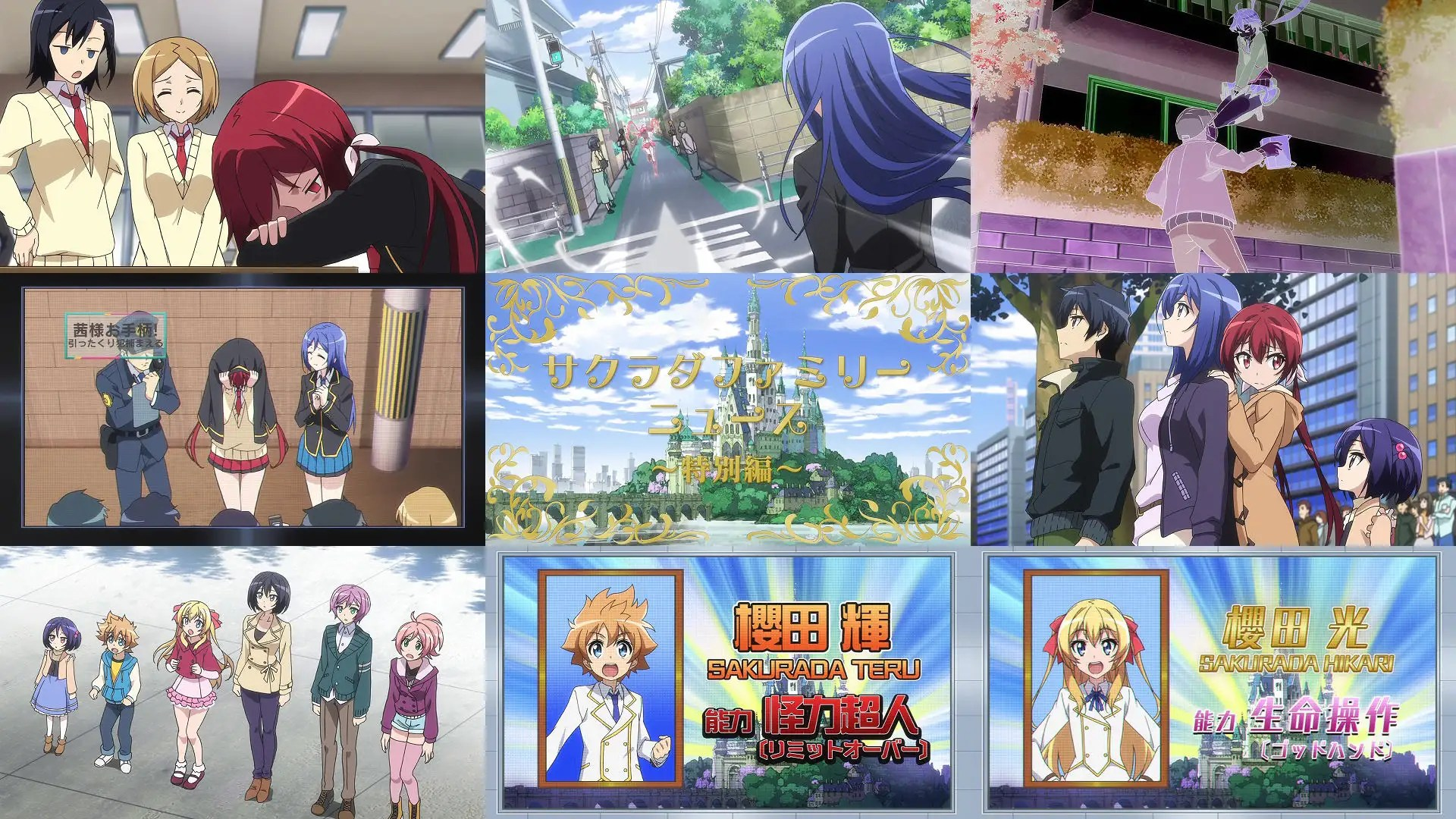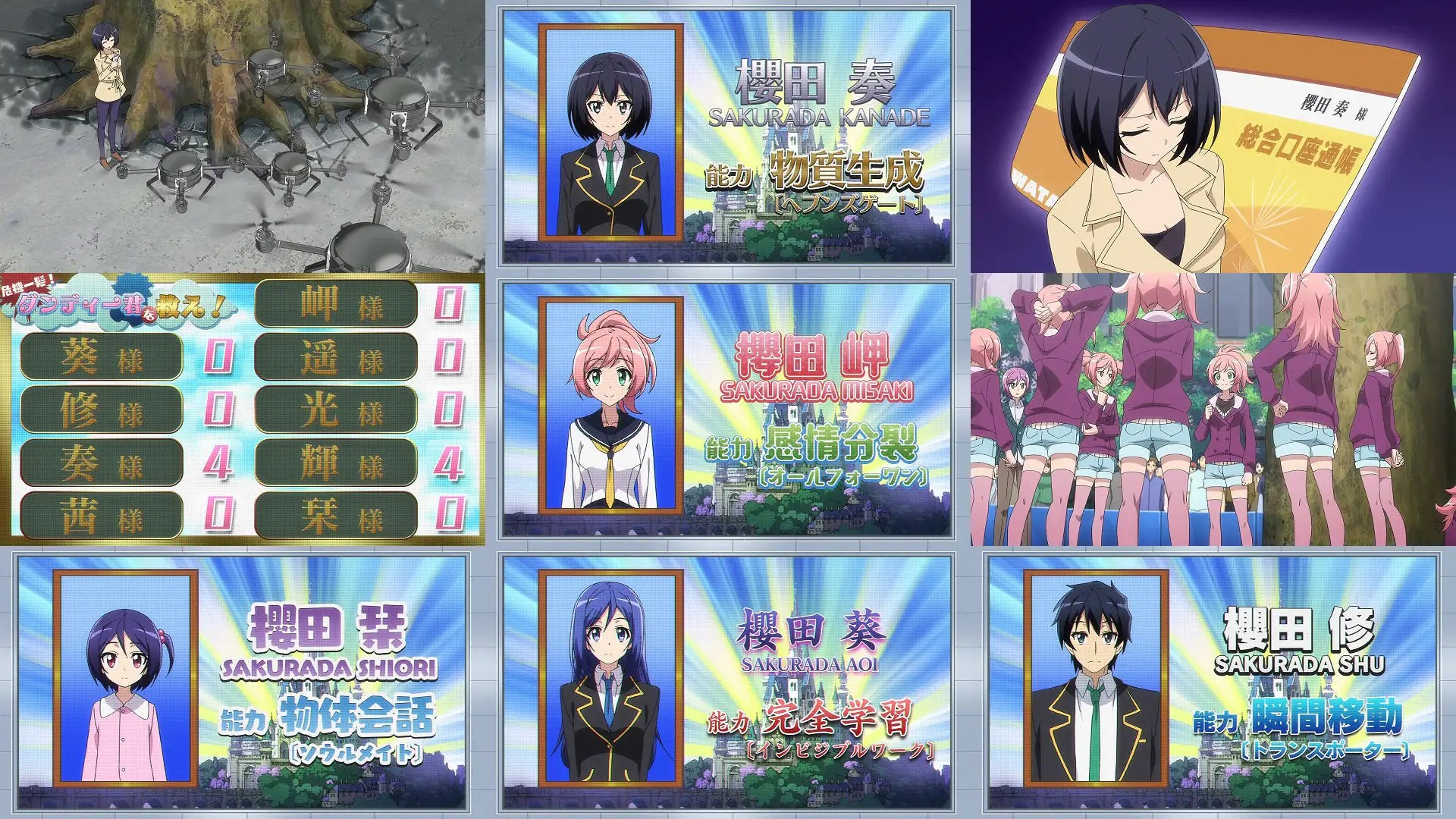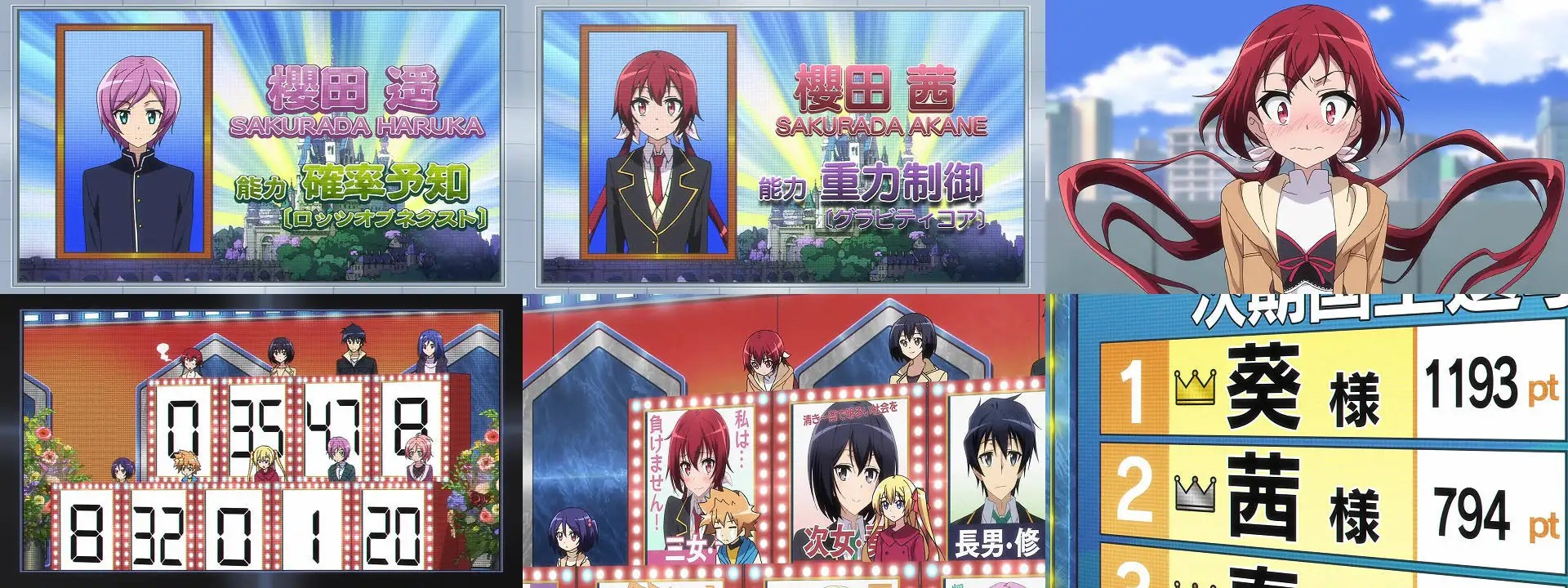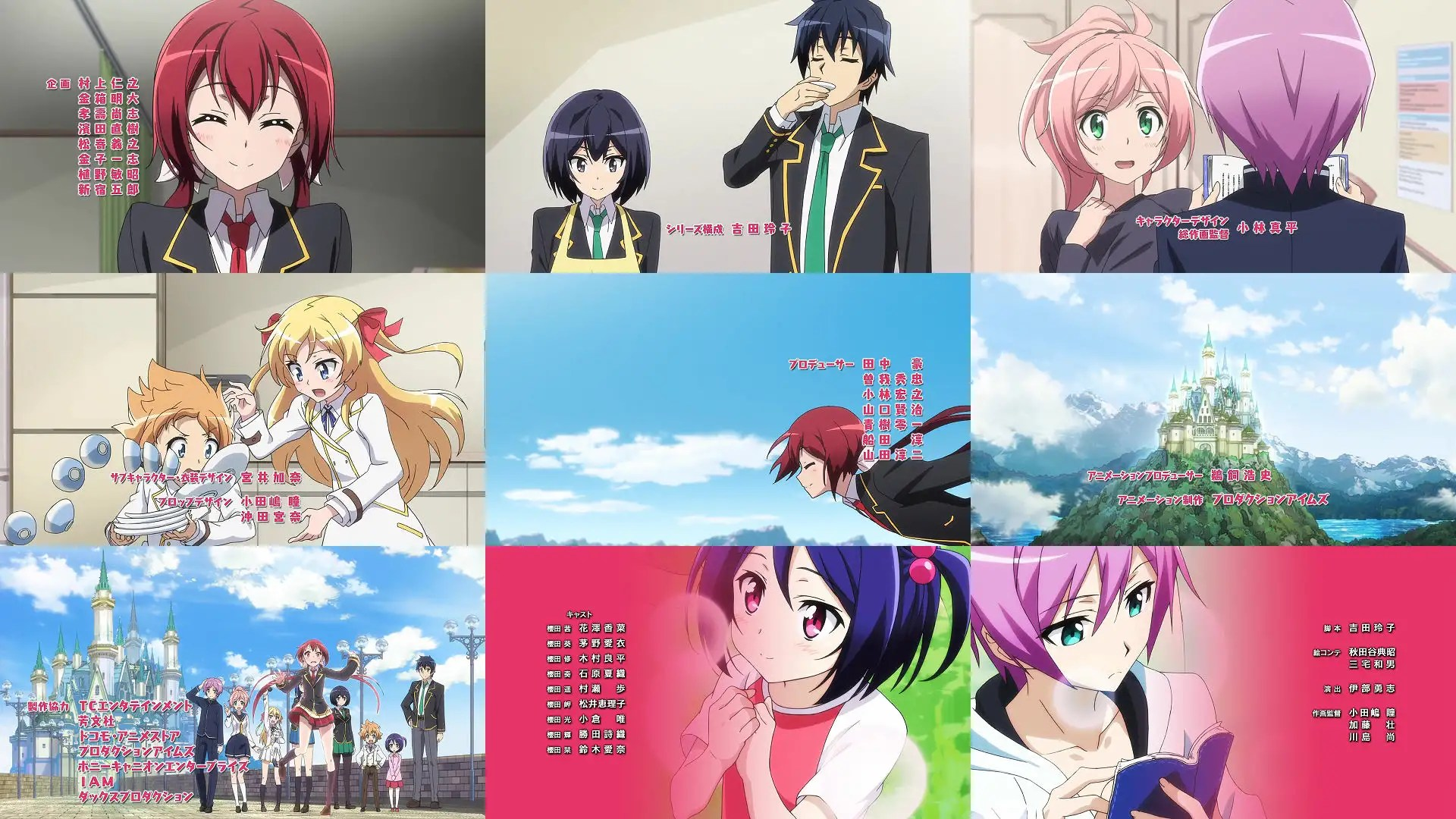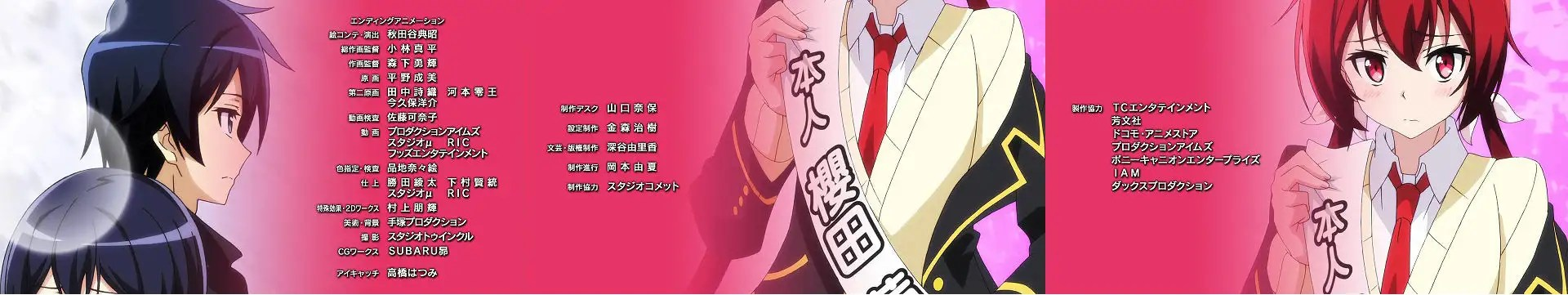4+3+3+4+3 3+3+3+3+3=32 「青春×機関銃 001」是個男裝麗人女主角，外觀上看根本就只是一個白白淨淨的男人。個人覺得她唯一的萌點，只有句句重複自己的姓氏這種三十年前的萌點而己。她不是明明只射了不到十發嗎？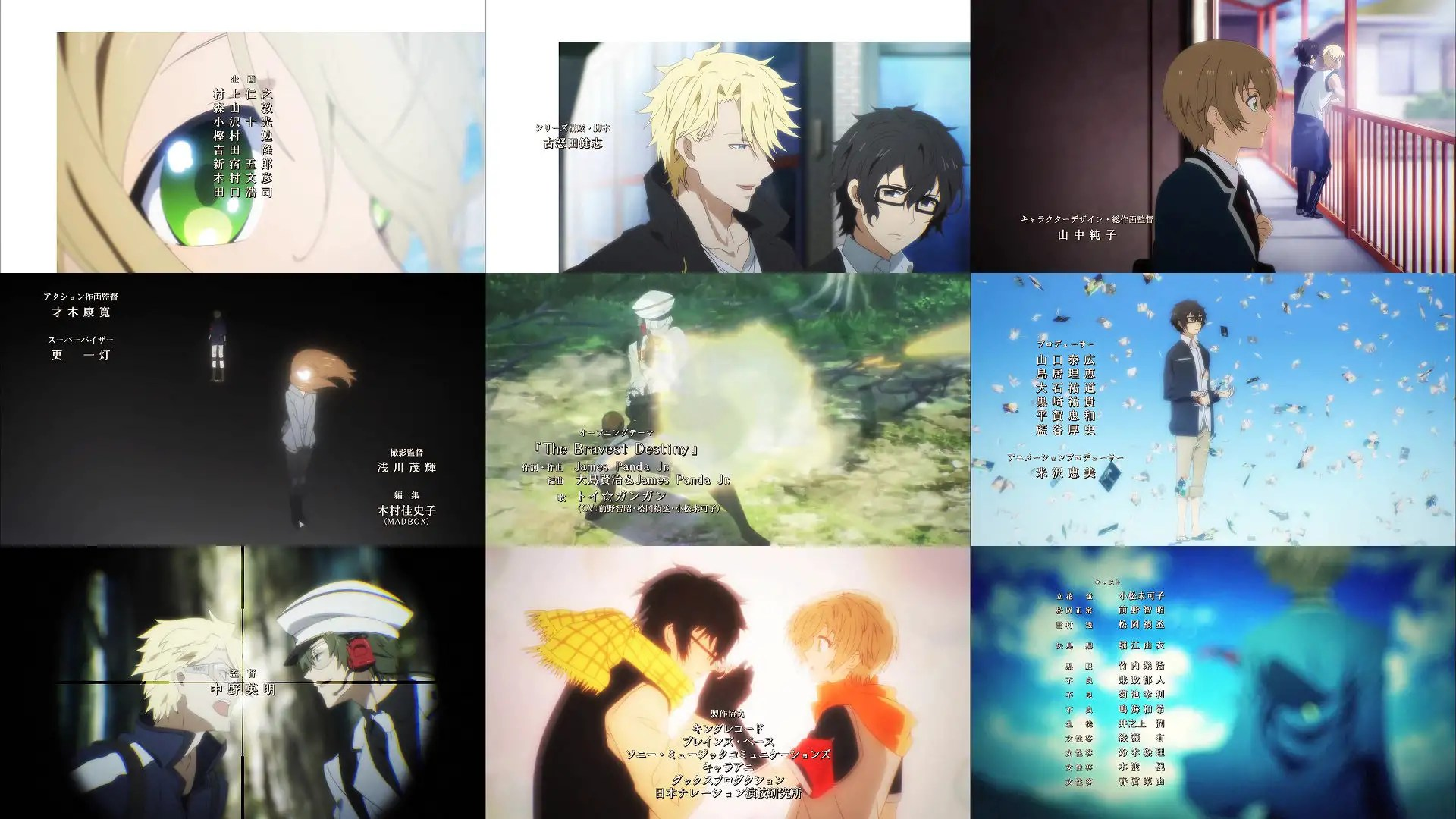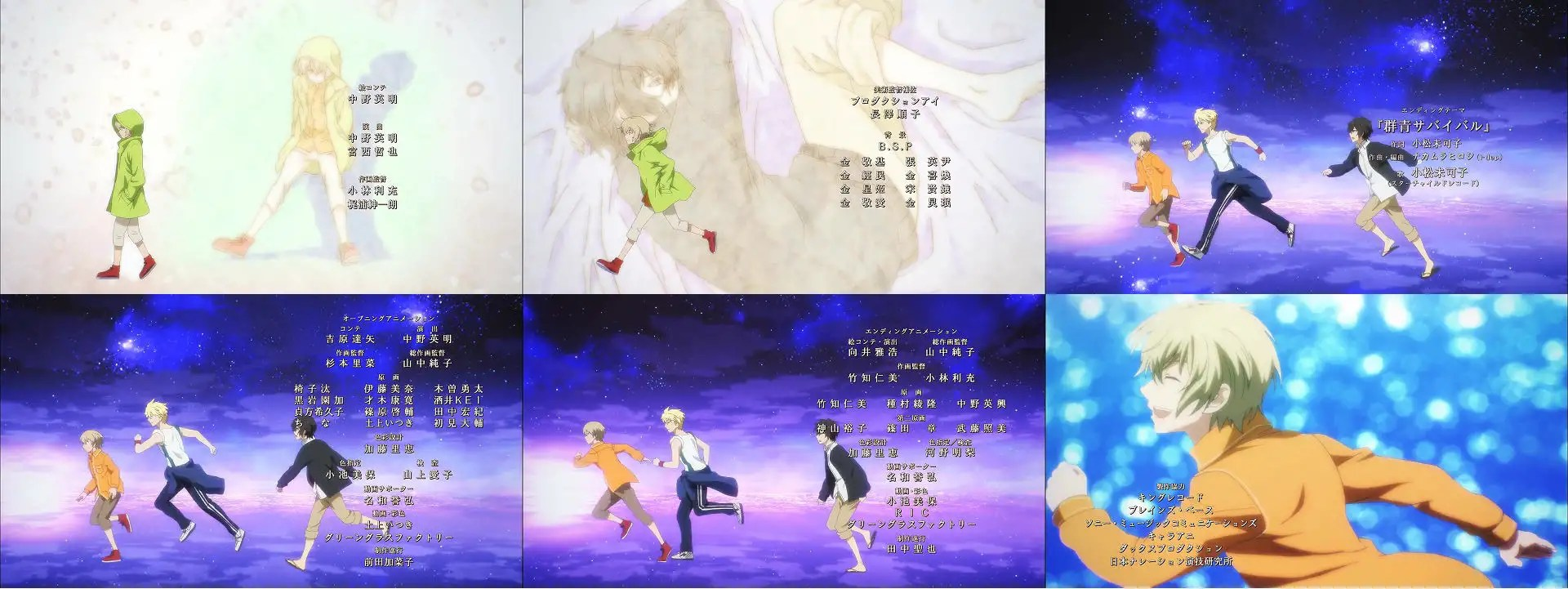4+4+3+3+3 3+3+3+4+3=33 「乱歩奇譚 Game of Laplace 001」主角群認識回。4+3+4+5+5 3+4+4+3+4=39 「六花の勇者 001」命運引導回。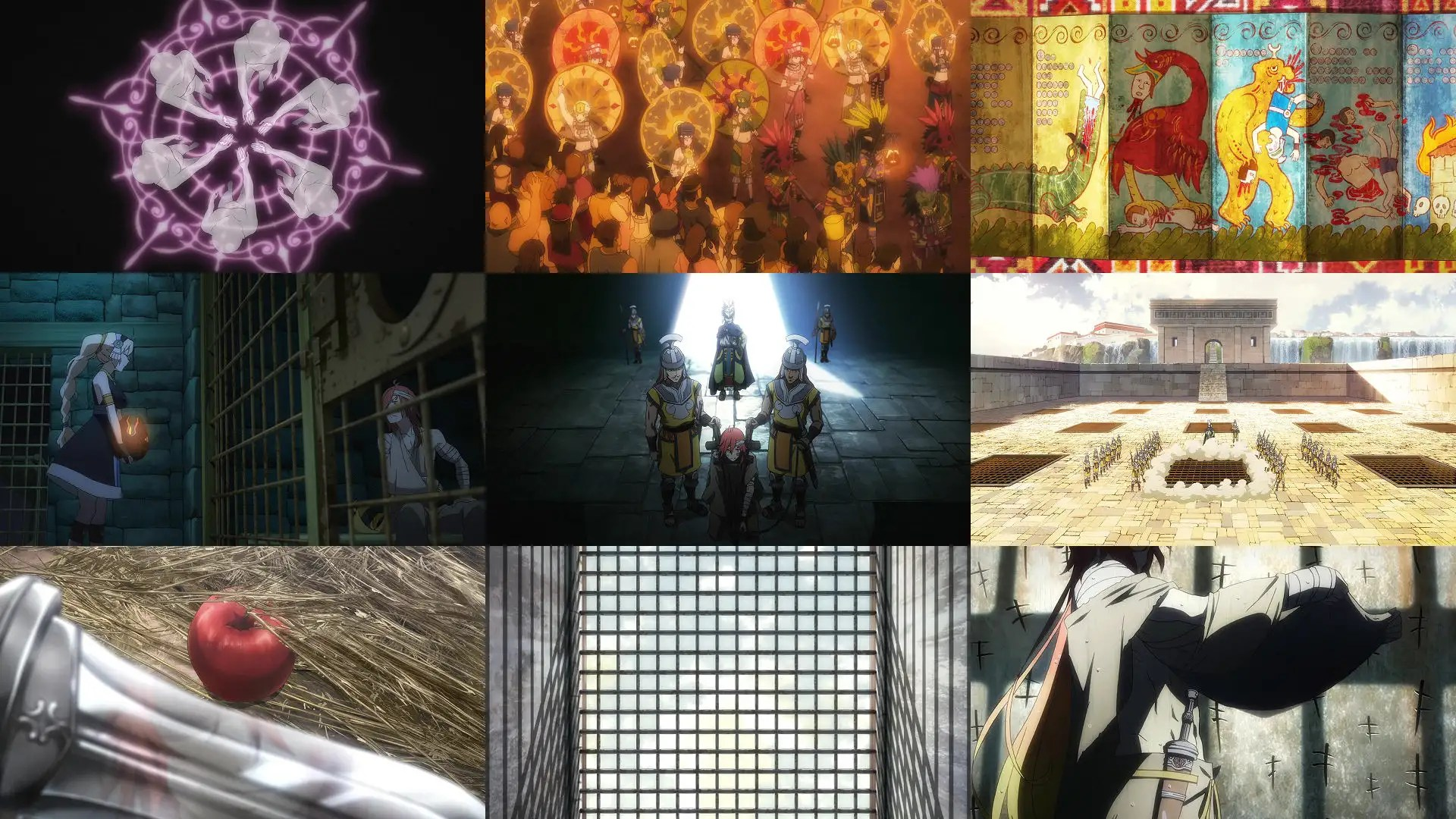4+3+3+4+4 4+3+3+3+4=35 「下ネタという概念が存在しない退屈な世界 001」 這片記得要收AT-X，比預期的好笑。故事及角色、後藤沙里緒的角色及下ネタ版涼宮令個人頗感興趣。故J項+1分。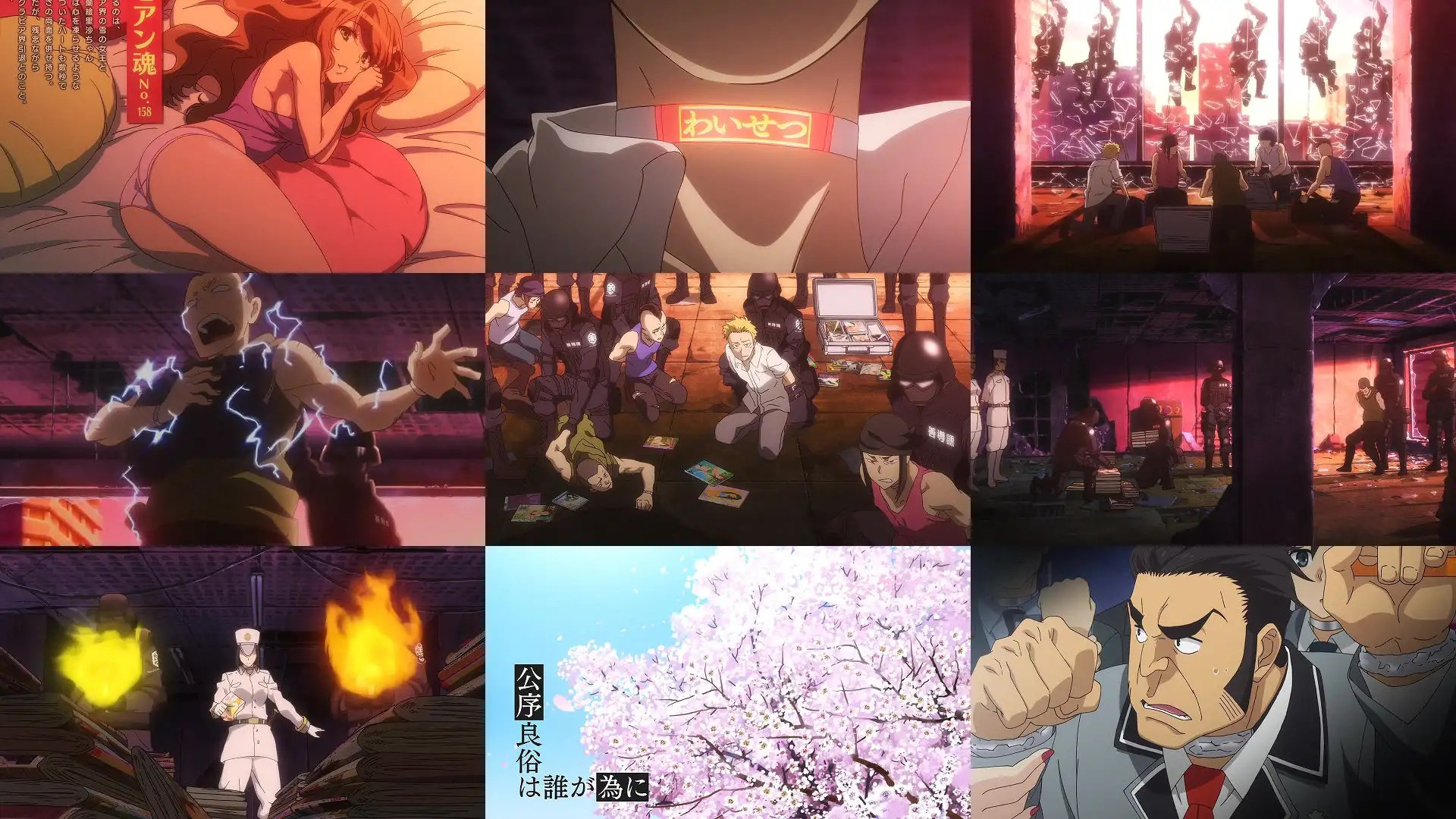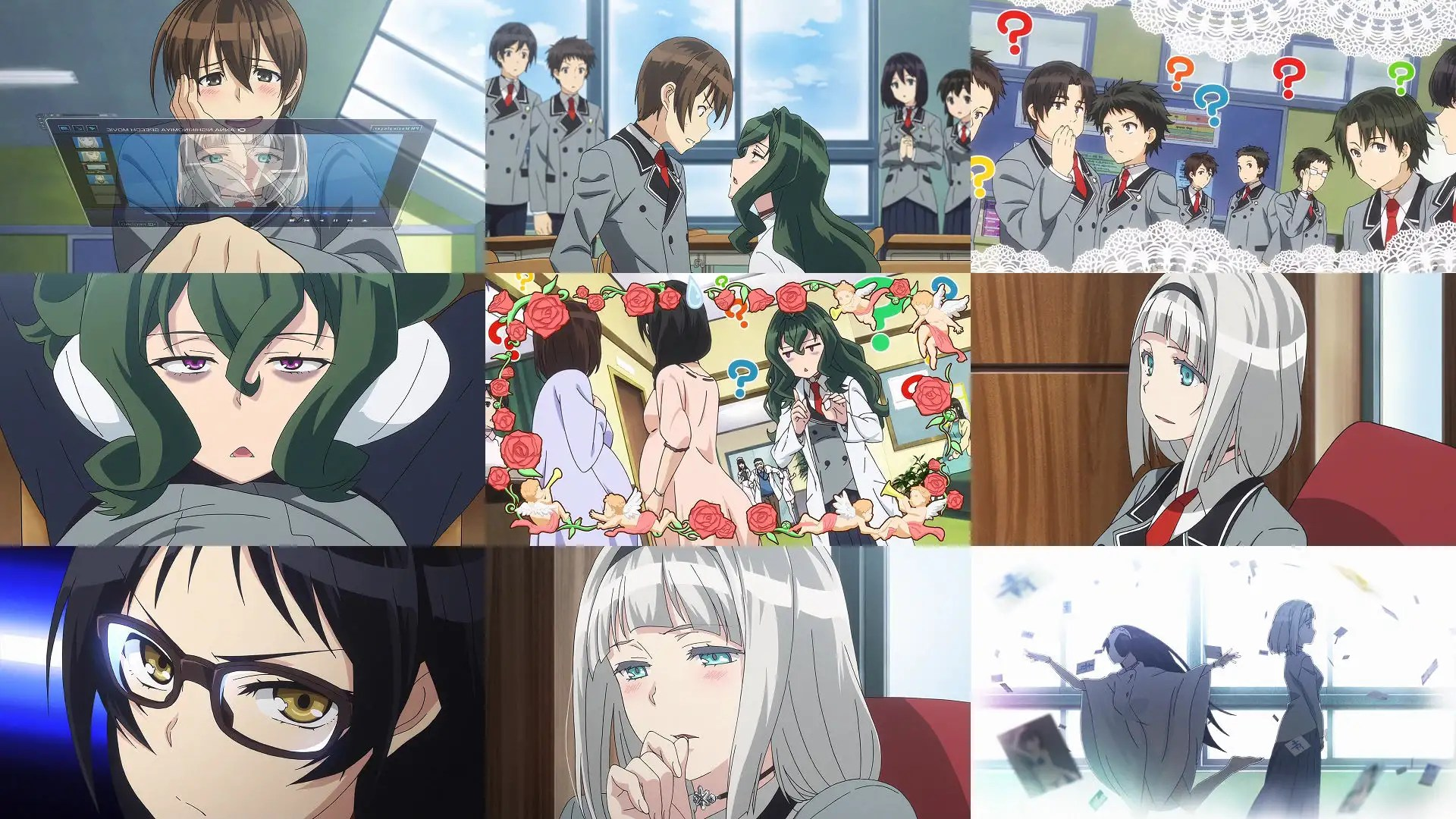5+4+4+5+5 4+4+4+3+3=41 「 Charlotte 001」前半社會，後半家族。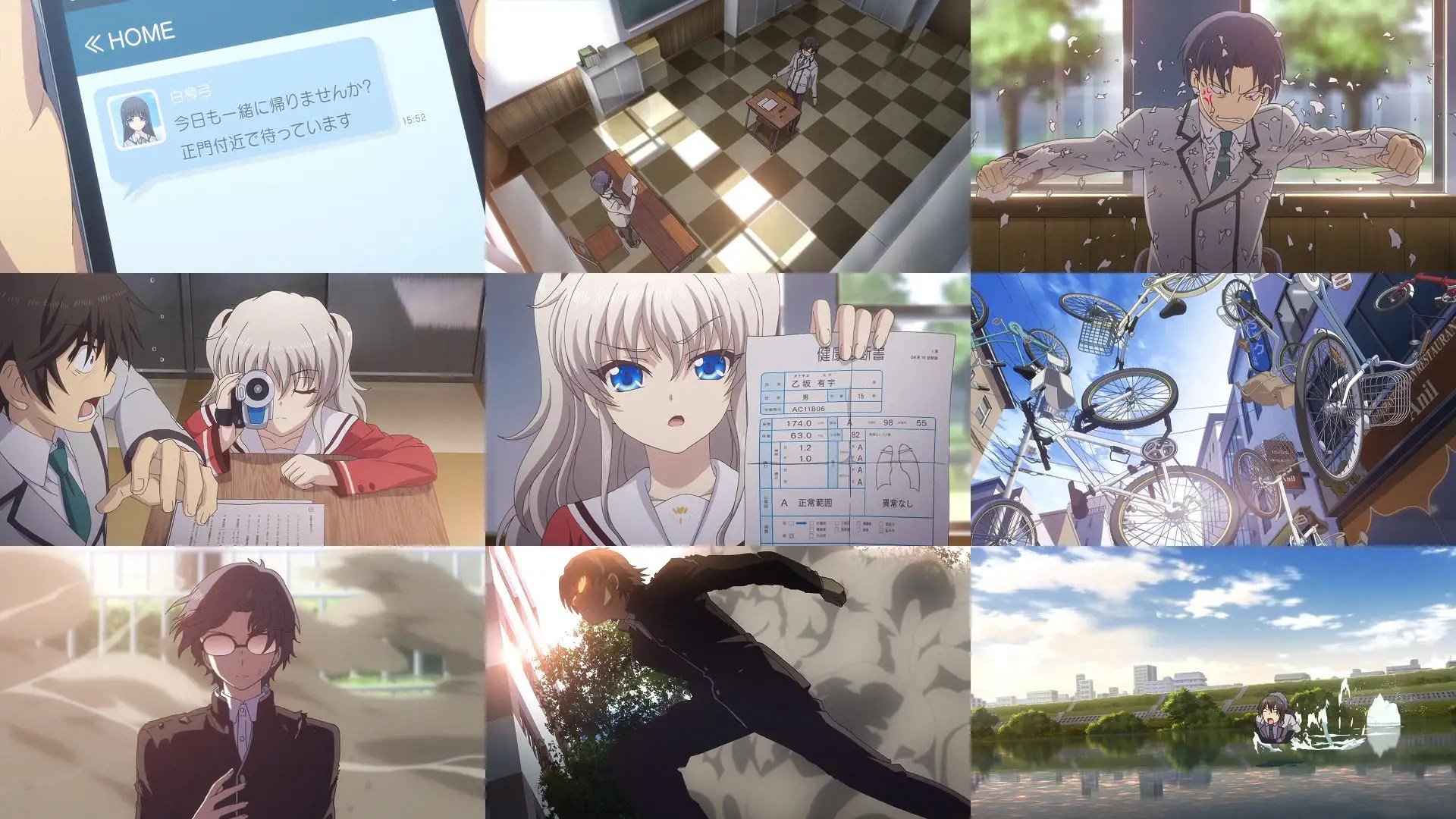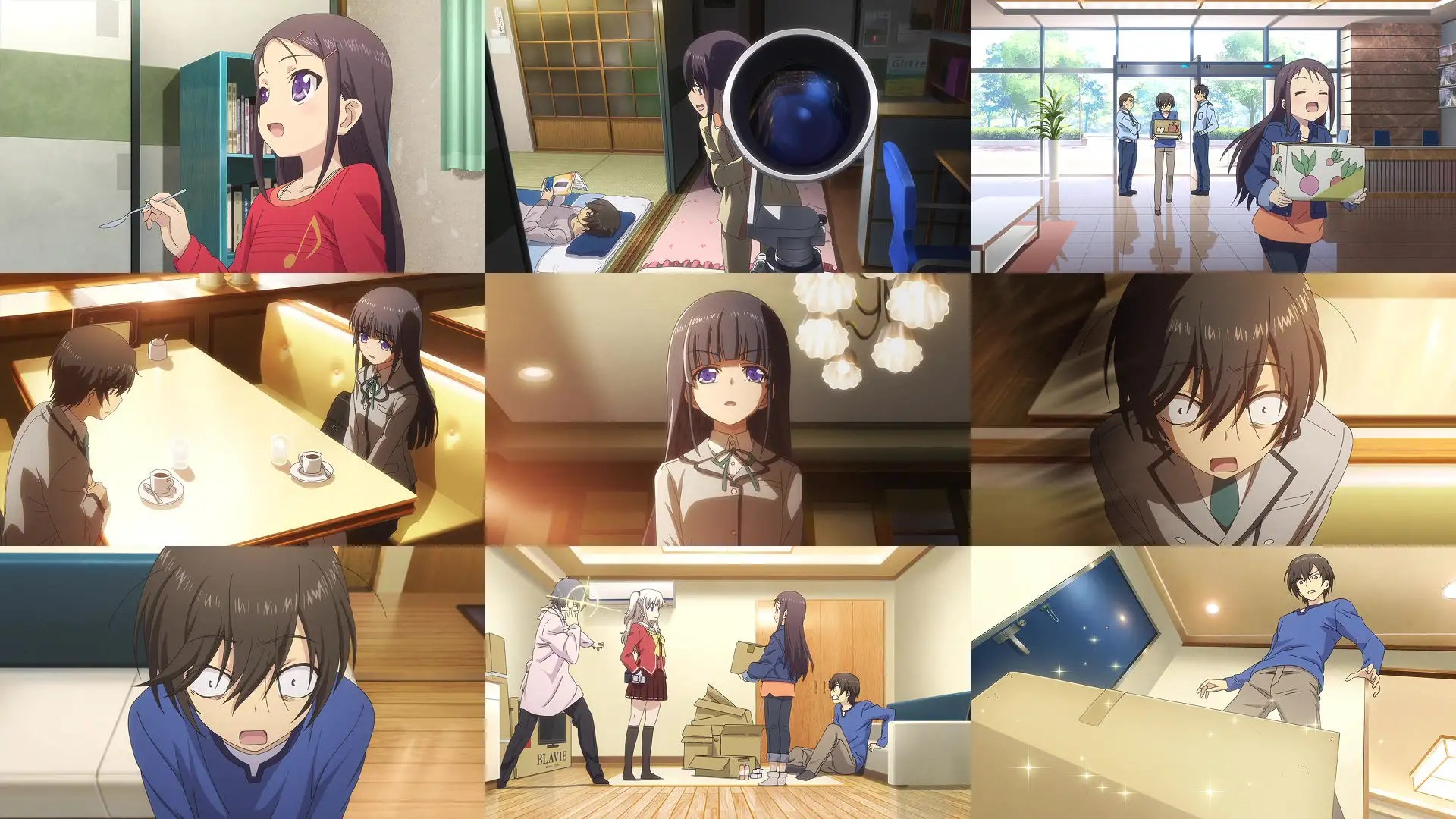3+3+3+3+3 4+4+3+3+3=32 「GATCHAMAN CROWDS insight」新成員介紹及宇宙和平使者回。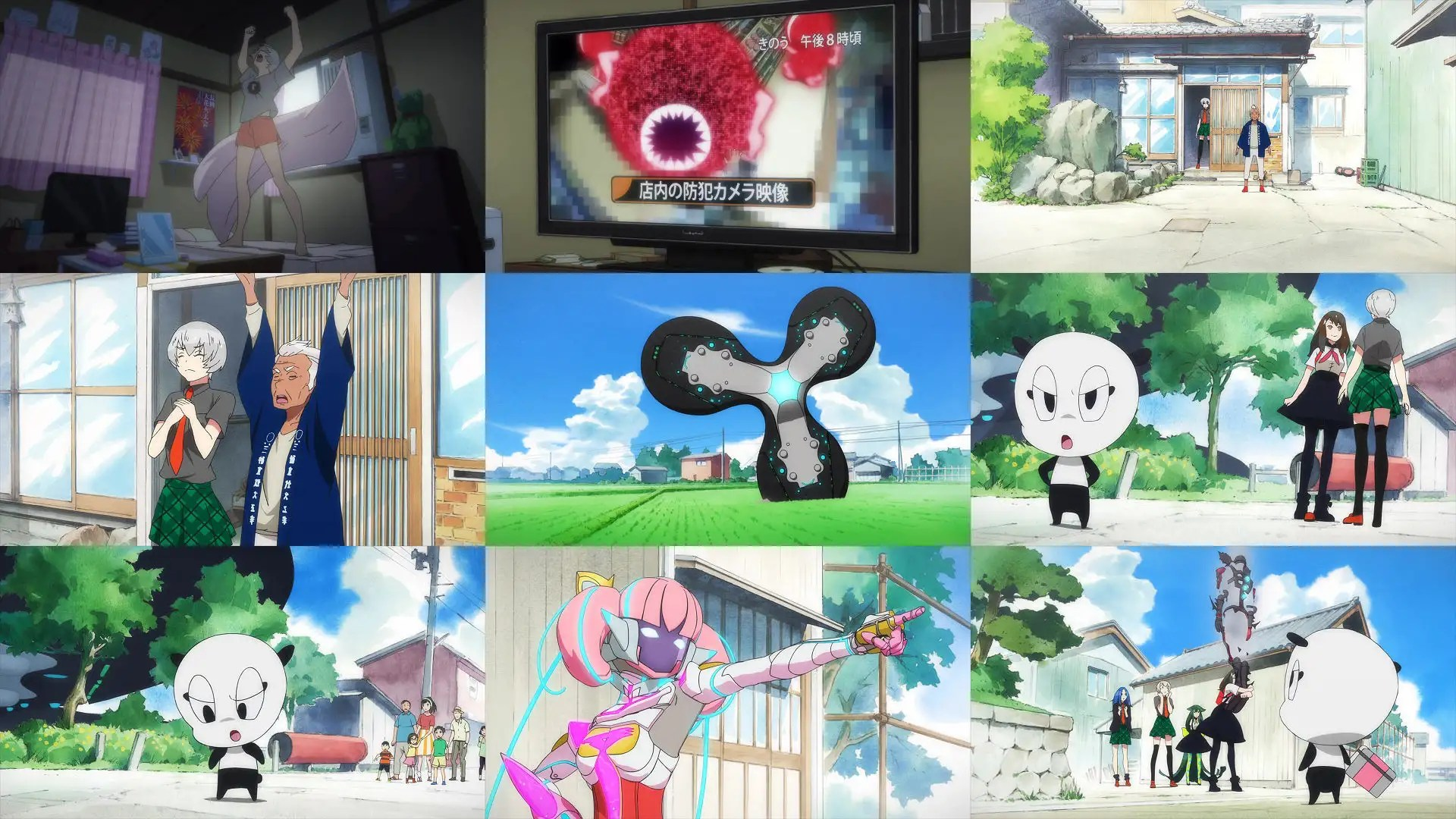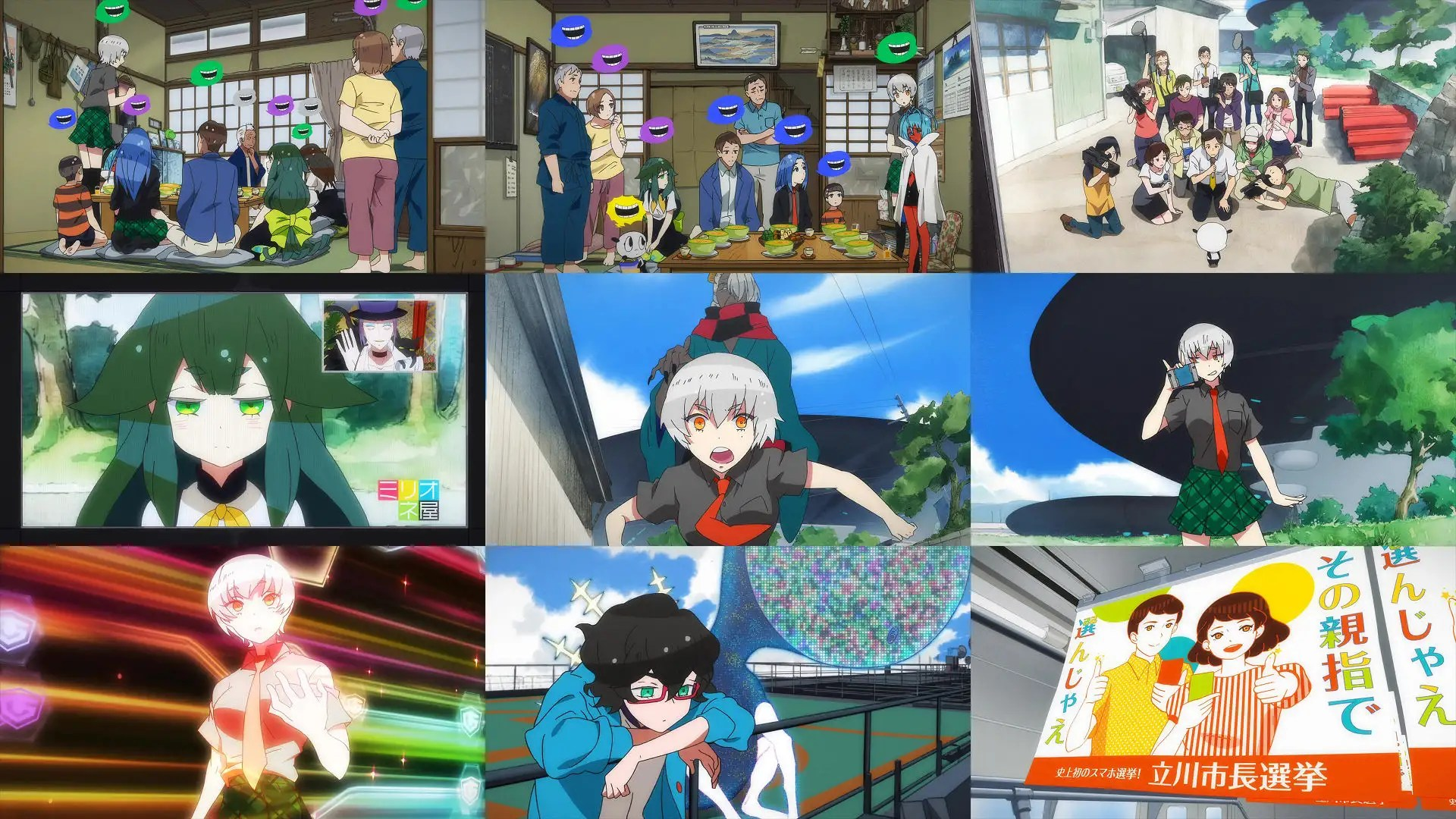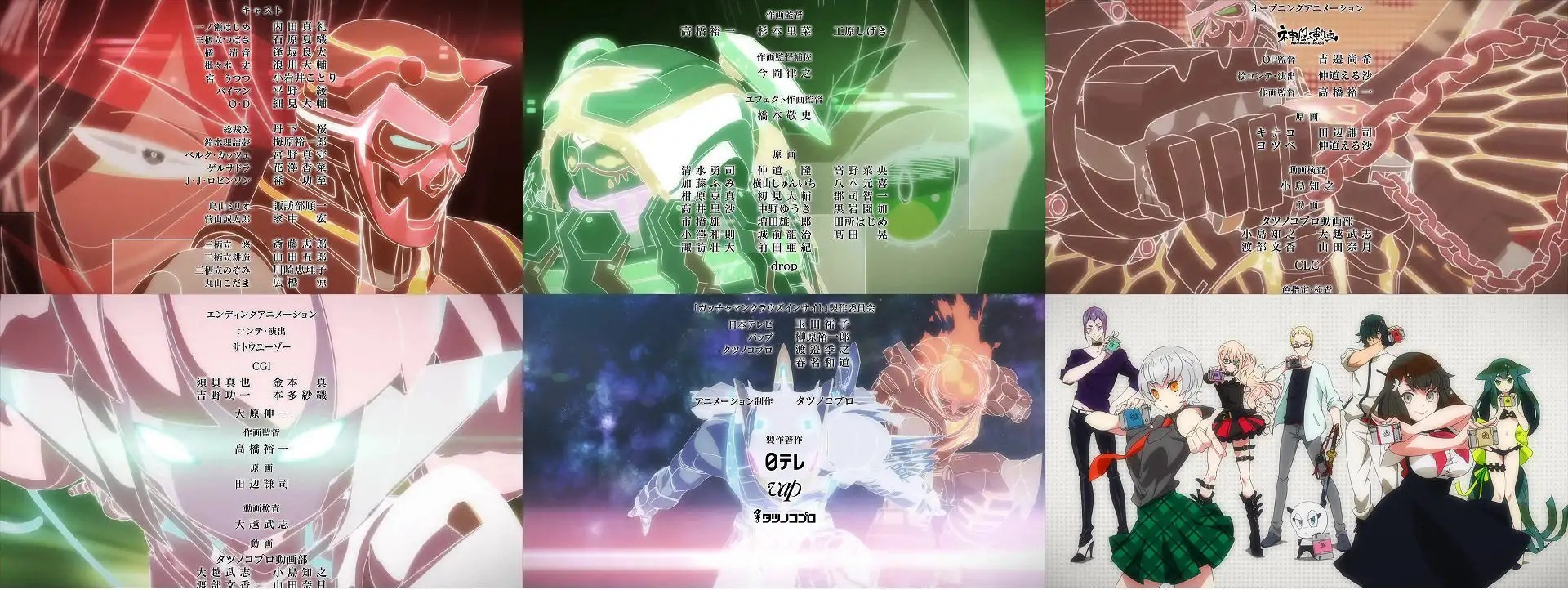3+3+3+3+3 4+4+3+3+3=32 「WORKING!!! 001」室內外音效距離感，聲優適性佳。

スクウェア・エニックスヤングガンガン」 每月大約也只有10萬部印刷 「咲-Saki-」「WORKING!!」「荒川アンダー」「夏色のキセキ」「緋彈のアリアAA」「天体戰士サンレッド」都是連載作品，有個強大的出版商在背後撐腰、真是幸福。